### Home > INT3 > Chapter 12 > Lesson 12.2.2 > Problem12-94

12-94.
1.This problem is a checkpoint for periodic functions. It will be referred to as Checkpoint 12.

2. For each trigonometric function determine the amplitude and period of the graph and sketch at least two cycles of the graph. Describe the graph as it relates to the parent function. Homework Help ✎

1. y = 2 sin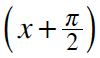2. y = cos(2x) – 1

3. y = tan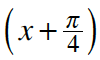3. Write an equation for each of the following graphs.

1.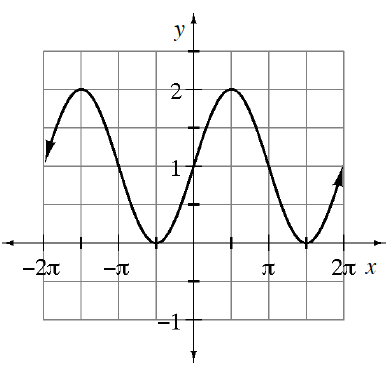2.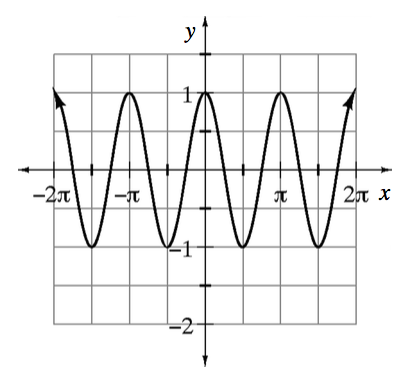4. Check your answers by referring to the Checkpoint 12 materials located at the back of your book.

5. Ideally, at this point you are comfortable working with these types of problems and can solve them correctly. If you feel that you need more confidence when solving these types of problems, then review the Checkpoint 12 materials and try the practice problems provided. From this point on, you will be expected to do problems like these correctly and with confidence.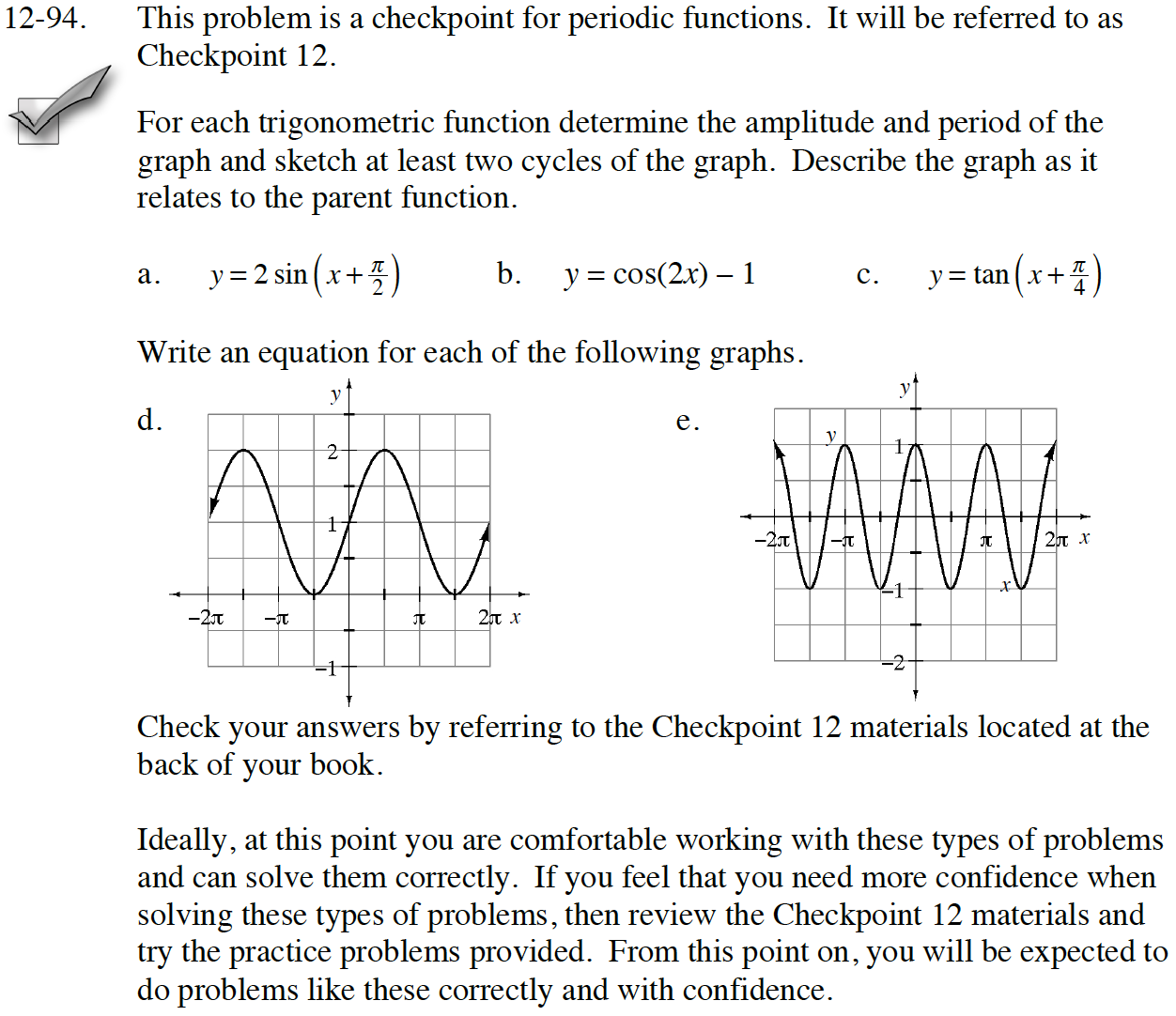Answers and extra practice are located in the back of your printed textbook or in the Reference Tab of your eBook.
If you have an eBook for INT3, login and then click the following link: Checkpoint 12 Answers and Practice